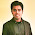## Course Schedule II

There are a total of n courses you have to take, labeled from 0 to n - 1.
Some courses may have prerequisites, for example to take course 0 you have to first take course 1, which is expressed as a pair: [0,1]
Given the total number of courses and a list of prerequisite pairs, return the ordering of courses you should take to finish all courses.
There may be multiple correct orders, you just need to return one of them. If it is impossible to finish all courses, return an empty array.
For example:
2, [[1,0]]
There are a total of 2 courses to take. To take course 1 you should have finished course 0. So the correct course order is [0,1]
4, [[1,0],[2,0],[3,1],[3,2]]
There are a total of 4 courses to take. To take course 3 you should have finished both courses 1 and 2. Both courses 1 and 2 should be taken after you finished course 0. So one correct course order is [0,1,2,3]. Another correct ordering is[0,2,1,3].
Note:
The input prerequisites is a graph represented by a list of edges, not adjacency matrices. Read more about how a graph is represented.

### Analysis:

This is highly related to the previous question Course Schedule. You can find all the details in this previous post and the only difference in this question is to add an array storing the results. The straightforward  way is to modify the BFS algorithm.

### Code(C++, BFS):

class Solution {
public:
vector<int> findOrder(int numCourses, vector<pair<int, int>>& prerequisites) {
int plen = prerequisites.size();
vector<int> ind(numCourses,0); //indegree vector
vector<int> res;

//construct map & get indegree of each vertex
vector<vector<bool> > map(numCourses, vector<bool>(numCourses, false));
for (int i=0;i<plen;i++){
//Important: in case of duplicates in prerequisites, only +1 indegree
if (map[prerequisites[i].first][prerequisites[i].second] == false){
map[prerequisites[i].first][prerequisites[i].second] = true;
ind[prerequisites[i].first]++;
}
}

//BFS
stack<int> st;
for (int i=0;i<numCourses;i++){
if (ind[i]==0) st.push(i);
}

int count = 0;  // to get the bool result

while (!st.empty()){
int tmp = st.top();
res.push_back(tmp);
st.pop();
count ++;
for (int i=0;i<numCourses;i++){
if (map[i][tmp]){
ind[i]--;
if (ind[i]==0) st.push(i);
}
}
}
if (count < numCourses){
vector<int> emp; // empty array
return emp;
}
else{
return res;
}
}
};


### Code(Python, BFS):

class Solution:
# @param {integer} numCourses
# @param {integer[][]} prerequisites
# @return {integer[]}
def findOrder(self, numCourses, prerequisites):
map = [[] for x in range(numCourses)]
ind = [0 for x in range(numCourses)]
res = []
for p in prerequisites:
if p not in map[p]:
ind[p] += 1
map[p].append(p)
st = []
for i in range(numCourses):
if ind[i] == 0:
st.append(i)
count = 0
while st:
tmp = st
st.pop(0)
res.append(tmp)
count += 1
for i in map[tmp]:
ind[i] -= 1
if ind[i] == 0:
st.append(i)

if count < numCourses:
return []
else:
return res



1.2.3.